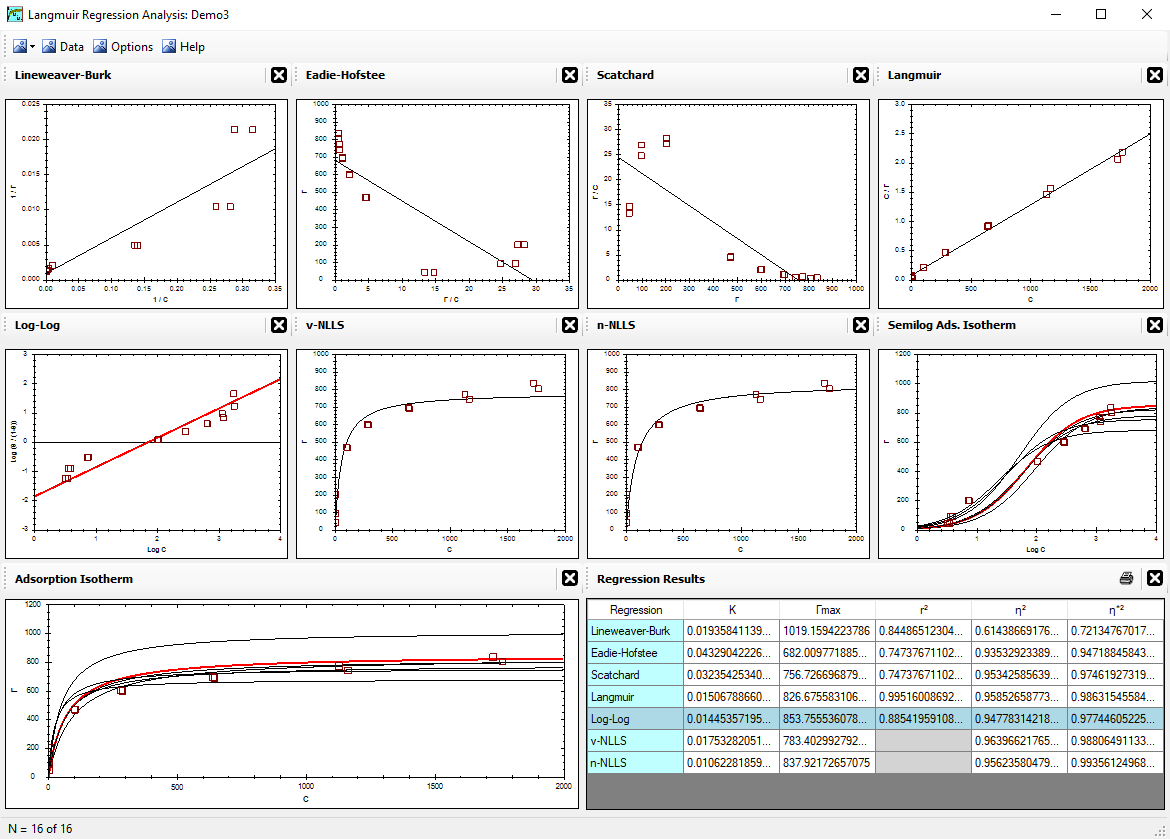Alfisol, Item 003: LMMpro, version 2.0 The Langmuir Optimization Program plus The Michaelis-Menten Optimization ProgramNLLS Regressions v-NLLS Optimization n-NLLS Optimization Axes Conversion Factor (ACF) Types of Errors

## Axes Conversion Factor (ACF)

The Axes Conversion Factor (ACF) describes the relative importance (weight) of an error in x versus an error in y. IF ACF = 1, then x axis and y axis values have the same weight. A large ACF value (>1) means that the y axis values are weighed more strongly. A small ACF value (<1) means that the x axis values are weighed more strongly.

The x-axis and y-axis values are sometimes not determined completely independent of each other. This is easy to illustrate with adsorption experiments involving a compound (A) in the liquid phase adsorbing to a solid phase. Typically, the total concentration of compound A in the mixture is known. It is then an easy calculation to determine the amount of compound A that was adsorbed by the solid phase (Γ) based on the amount of compound A remaining in the liquid phase at equilibrium (c). That is, Γ is not actually measured, but rather it is extrapolated from c. Hence, any error in the measurement of c will also express a similar error in Γ. Although there is no definitive proof for how the ACF value should be determined, we can use this relationship (or dependency of the x,y data to estimate the ACF value. An example follows that illustrates a useful procedure for calculating the ACF value.

Example for estimating the ACF value:

• Assume an adsorption experiment in a closed container. This is a batch reaction type of experiment, where the amount adsorbed by the solid is a function of the amount in solution at equilibrium.
• Assume that the data collected are such that the y-axis units are μg m-2.
Assume that the data collected are such that the x-axis units are mg L-1.
• The question asked here is: "one unit change in y equals how many units change in x?"
• Assume solids concentration = 9.24 g L-1.
• Assume specific surface area of the solid = 100 m2 g-1.
• Given the above conditions:
 1 μg m2 × 100 m2 g × 9.24 g L × mg 1000 μg = 0.924 mg L
• For this example, ACF = 0.924 .

What does ACF mean?
It will carry a different meaning with every application. In the adsorption example above, ACF = 0.924. Notice that the amount adsorbed was determined based on the amount remaining in solution. This practice is common in adsorption isotherm experiments, where the y-axis values are not truly independent of the x-axis values. A loss of 0.924 mg/L from the liquid phase is equal to an adsorption amount of 1 μg/m2. An error of 0.924 mg/L for the liquid phase concentration is equal to an adsorption error of 1 μg/m2. Arithmetically, nothing really changes if you determine the amount adsorbed by direct measurement of the solid phase because the adsorption value is directly related to the liquid phase equilibrium value as a result of the principle of mass balance. That is, the total sum of the nonadsorbed liquid portion plus the adsorbed solid portion must be constant at all times.

We can apply a similar example to enzyme kinetics. In enzyme kinetics studies, the rate of reaction (v) can be evaluated based on the rate of loss of substrate (S). Accordingly, an error in the measurement of S will result in an error in the measurement of v. For example, an easy analytical error to encounter involves the instrumental calibration for substrate concentration, where a blank is correctly set to zero but a standard high value is slightly off. Now if a calibration error results in a 5% overestimate in substrate concentration at high concentrations but a correct value at low concentrations (such as at time 0 and time t), then you will also have a 5% error in the reaction rate when expressed as unit change in concentration per unit change in time. The ACF value measures this error relationship. Arithmetically, nothing really changes if you determine the reaction rate by direct measurement of the product formed because the product concentration is directly related to the reactant concentration as a result of the principle of mass balance. That is, the total sum of the product formed plus the reactant remaining must be constant at all times.

Note, however, that there is presently no definitive definition for ACF. Accordingly, the ACF parameter can be used as a relative weight conversion factor of the axes, instead of a unit conversion factor. Changing the value of ACF gives the user more flexibility on how to interpret the regression results. The ACF value is not a unique value. A large ACF value (>1) means that the y-axis values are weighed more strongly, and a better fit is observed for the data with high [c] or [S] values. A small ACF value (<1) means that the x-axis values are weighed more strongly, and a better fit is observed for the data with low [c] or [S] values.

In principle, modification of the ACF value can be used to highlight sensitivity of the regression results toward deviations in the x-axis or y-axis data values. No sensitivity is observed when the data and theory are perfect. In spite of this interesting observation with modified ACF values, I recommend that only one ACF value be used with your data, and that it be calculated similarly to the one example illustrated above that resulted in ACF = 0.924. If alternate ACF values cannot be defended, then they should not be used in the final presentation of the results. That is, I recommend that the ACF value be used only as a unit conversion factor, and thus remain true to the experimental propagation-of-error from the x-axis to the y-axis values collected and displayed on the original plot.

<Reference = CTT-10>